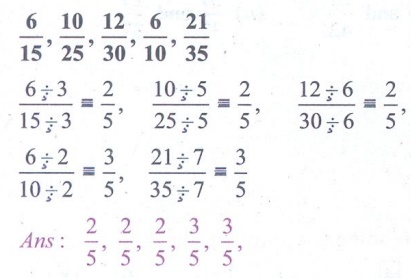Home | | Maths 5th Std | Exercise 6.2 (Fractions)

# Exercise 6.2 (Fractions)

Text Book Back Exercises Questions with Answers, Solution : 5th Maths : Term 3 Unit 6 : Fractions : Exercise 6.2 (Fractions)

Exercise 6.2

1. Write the suitable number in the box.2. Find an equivalent fraction with denominator 18 for each of the following fractions.1/2, 2/3,4/6,2/9,7/9,5/3

1/2 × 9/9 = 9/18, 2/3 × 6/6 = 12/18, 4/6×3/3 = 12/18

2/9 × 2/2 = 4/18, 7/9 ×2/2 =14/18, 5/3 ×6/6 = 30/18

Answer: 9/18, 12/18, 12/18, 4/18, 14/18, 30/18

3. Find an equivalent fraction with denominator 5 for each of the following fractions.6/15, 10/25, 12/30, 6/10, 21/35

6 ÷3 / 15÷3 = 2/5, 10 ÷5 / 25 ÷5 = 2/5, 12 ÷6 / 30 ÷6 = 2/5

6÷2 / 10÷2 = 3/5, 21÷7 / 35 ÷7 = 3/5

Answer: 2/5, 2/5, 2/5, 3/5, 3/5

Tags : Fractions | Term 3 Chapter 6 | 5th Maths , 5th Maths : Term 3 Unit 6 : Fractions
Study Material, Lecturing Notes, Assignment, Reference, Wiki description explanation, brief detail
5th Maths : Term 3 Unit 6 : Fractions : Exercise 6.2 (Fractions) | Fractions | Term 3 Chapter 6 | 5th Maths